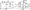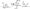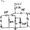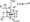# Analog Electronic Circuits July 2008

Note : Answer any FIVE full questions, selecting at least two questions from each part.

PART-A

1 a. Differentiate between static and dynamic resistance of a semi conductor diode.

b. Explain with the help of a circuit diagram the working of a Full Wave Rectifier. Derive expressions for i) Idc ii) Irms iii) Vdc iv) Ripple factor v) Rectifier efficiency.

c. For the circuit shown, in Fig.Q 1(c) write the transfer characteristic equations. Assume diodes are ideal. Plot Vo against Vi, indicating all slopes and voltage levels.2 a. Design a voltage divider bias circuit with Vcc – 10 V, Rc = 1.5 K ohm, IC – 2 mA, VCE – 5 V, β = 50. Assume silicon transistor and stability factor S = 5.

b. Derive an expression for the stability factor S(Ico) for a voltage divider bias circuit.

c. Determine Rb and Rc for the transistor inverter of Fig.Q2(c) if IcSAt -10 mA.3 a. For the network of Fig.Q3

(a): i) Determine re  ii) Calculate Zi and Z iii) Find Av Given β = 100 SI transistor.

b.  Draw the emitter follower circuit Derive expressions; for:

i)Zi ii) Zo iii) Av using re model.

c.   Define h-parameters. Draw the h-parameter model of a transistor.

4 a. Determine the lower cutoff frequency for the network of Fig.Q4(a). Given p = 100, r0 “ oo ohm. Determine the mid band gain. If Cbe – 36 pF, Cbc = 4 pF, Cw = 6 pF, C.v = 8 pF. Determine fH.and fH and sketch the frequency response for low and high w o ^^*0frequency regions using the results.b. Calculate the overall lower 3 db and upper 3 db frequencies for a 3 stage amplifier having an individual fj = 40 Hz and fz — 2 MHz.

PART-B

5 a. Draw the cascade configuration and list the advantages of this circuit.

b.  Determine A;, Ri; Av and Ro for the circuit shown in fig.Q5(b).

Given h parameters hie = 1.1 k ohm, hre = 2 x 10″4, hoe – 25 x 1 O’6 U, hfe = 50.

c.  List the advantages of negative feedback amplifier. Derive expressions for Zjf and Z0f for voltage series feedback amplifier.

6 a. Explain the working of a class B push pull amplifier. Prove that the maximum efficiency is 78.5%.

b.  A single transistor amplifier with transformer coupled load produces harmonic amplitudes in the output as Bo – 1.5 mA, Bi – 120 in A, B2 – 10 mA, 83-4 mA, B4 = 2 mA, B5 – 1 mA.

i) Determine the percentage total harmonic distortion

ii) Assume second identical transistor is used along with suitable transformer to provide push pull operation. Using the above harmonic amplitudes, determine the new total harmonic distortion.

7 a. Explain with the help of a circuit diagram, the working of an RC phase shift oscillator.

b.  With the help of Barkhousen criterion, explain the working of a BJT crystal oscillator.

Calculate the frequency of a Wien Bridge oscillator circuit when R = 12 k ohm and C = 2400 pf.

8 a. Determine Zh Zq and Av for the circuit shown in Fig.Q8(a), if Yfs = 3000 }is and= 50 jxs.Fig. Q8(a)

b.  Determine Zi, Zo , and Av if rd= 40 k£l for fig.Q 8(b).c.  With the help of circuits and equations, show different biasing arrangements for depletion type MOSFET.